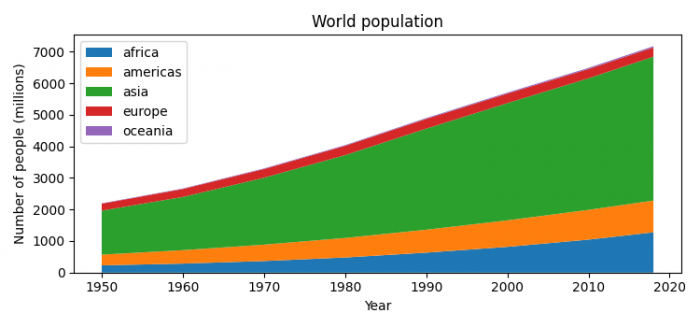# How to create a 100% stacked Area Chart with Matplotlib?

To create a 100% stacked Area Chart with Matplotlib, we can take the following steps −

• Set the figure size and adjust the padding between and around the subplots.

• Create a list of years.

• Make a dictionary, with list of population in respective years.

• Create a figure and a set of subplots.

• Draw a stacked Area Plot.

• Place a legend on the figure, at the location ''upper left''.

• Set the title, xlabel and ylabel.

• To display the figure, use show() method.

## Example

import matplotlib.pyplot as plt

plt.rcParams["figure.figsize"] = [7.50, 3.50]
plt.rcParams["figure.autolayout"] = True

year = [1950, 1960, 1970, 1980, 1990, 2000, 2010, 2018]

population_by_continent = {
'africa': [228, 284, 365, 477, 631, 814, 1044, 1275],
'americas': [340, 425, 519, 619, 727, 840, 943, 1006],
'asia': [1394, 1686, 2120, 2625, 3202, 3714, 4169, 4560],
'europe': [220, 253, 276, 295, 310, 303, 294, 293],
'oceania': [12, 15, 19, 22, 26, 31, 36, 39],
}

fig, ax = plt.subplots()
ax.stackplot(year, population_by_continent.values(), labels=population_by_continent.keys())

ax.legend(loc='upper left')
ax.set_title('World population')

ax.set_xlabel('Year')
ax.set_ylabel('Number of people (millions)')

plt.show()

## Output